# Python多任务之线程

## 多任务介绍import time

def sing():
for i in range(5):
print("我喜欢唱")
time.sleep(1)

def dance():
for i in range(5):
print("我喜欢跳")
time.sleep(1)

def main():
sing()
dance()
pass

if __name__ == "__main__":
main()

我喜欢唱import time

def sing():
for i in range(5):
print("我喜欢唱歌")
time.sleep(1)

def dance():
for i in range(5):
print("我喜欢跳舞")
time.sleep(1)

def main():
t1.start()
t2.start()

if __name__ == '__main__':
main()

我喜欢唱歌

### 多任务

• 如果是多核cpu同时运行多个任务，我们就称之为并行，是真的多任务；任务数少于cpu数量；
• 如果是单核cpu切换着运行多个任务，我们就称之为并发，是假的多任务。任务数多于cpu数量；
• 但因为日常中，任务数一般多于cpu核数，所以我们说的多任务一般都是并发，即假的多任务；

2. 编写多任务所需要的的函数；
4. 调用实例对象的start方法，创建子线程。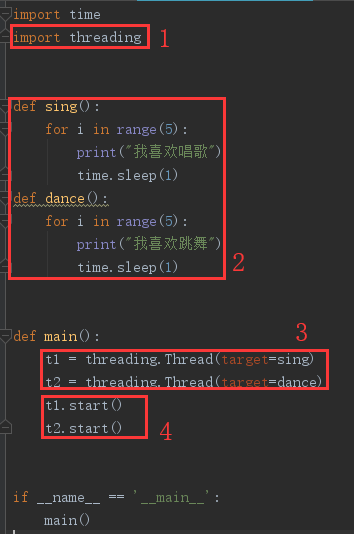import time

def sing():
for i in range(5):
print("我喜欢唱歌")
time.sleep(1)

def dance():
for i in range(5):
print("我喜欢跳舞")
time.sleep(1)

def main():
t1.start()
t2.start()

if __name__ == '__main__':
main()

• 函数名() 表示函数的调用
• 函数名 表示使用对函数的引用，告诉函数在哪；

#### 代码解读

def main():
t1.start()
t2.start()

t1 = threading.Thread(target=sing)

t1.start()

### 多线程创建执行理解

threading.enumerate()

#### 让某些线程先运行import time

def sing():
for i in range(5):
print("-----sing----%d" % i)

def dance():
for i in range(5):
print("-----dance----%d" % i)

def main():
t1.start()
time.sleep(1)
print("sing")

t2.start()
time.sleep(1)
print("dance")

if __name__ == '__main__':
main()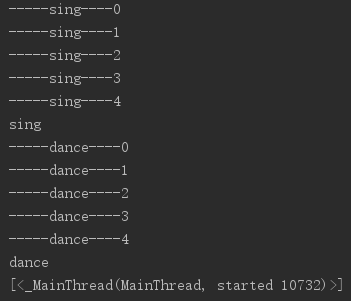#### 循环查看当前运行的线程数import time

def sing():
for i in range(5):
print("-----sing--%d--" % i)
time.sleep(1)

def dance():
for i in range(5):
print("-----dance--%d--" % i)
time.sleep(1)

def main():
t1.start()
t2.start()
while True:
# print("当前运行线程数：%s" % t_len)
if t_len <= 1:
break
time.sleep(1)

if __name__ == '__main__':
main()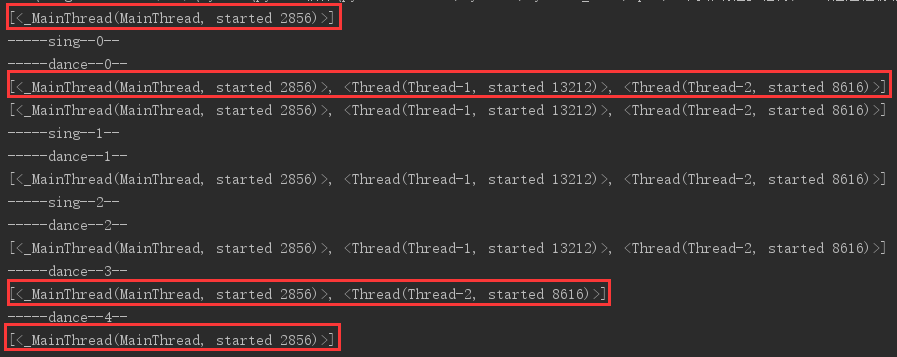#### 验证子线程的执行时间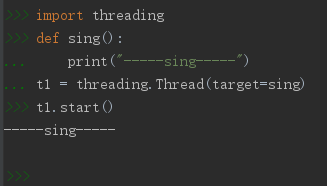import threading
def sing():
print("-----sing-----")
t1.start()
-----sing-----

#### 验证子线程的创建时间import time

def sing():
for i in range(5):
print("----sing----")
time.sleep(1)

def main():
t1.start()

if __name__ == '__main__':
main()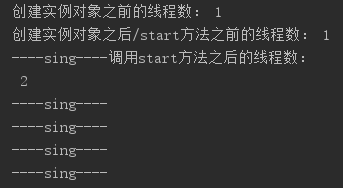3. 然后创建这个类的实例对象；
4. 调用实例对象的start方法，就创建了一个线程。import time

def run(self):
print("---run---")
for i in range(3):
msg = "我是%s,i--->%s" % (self.name, str(i))  # self.name中保存的是当前线程的名字
print(msg)
time.sleep(1)

def main():
t1.start()

if __name__ == '__main__':
main()

---run---

• 这种方法适用于一个线程里面要做的事情比较复杂，要封装成几个函数来做，那么我们就将它封装成一个类。
• 在类中定义其他的几个函数，可以在run里面进行调用这几个函数。
• 创建线程时使用哪种方法比较好？哪个简单使用哪个。

## 多线程共享变量

#### 验证多线程中共享全局变量

定义一个全局变量，在函数1中加1，在函数2中查看，让线程控制的函数1先执行，如果线程函数2的查看结果和函数1的查看结果一样，那么就证明多线程之间共享全局变量。import time

g_num = 100

def sing():
global g_num
g_num += 1
print("---sing中的g_num: %d---" % g_num)
time.sleep(1)

def dance():
print("---dance中的g_num: %d---" % g_num)
time.sleep(1)

def main():
t1.start()
t2.start()
print("---主线程中的g_num: %d---" % g_num)

if __name__ == '__main__':
main()

---sing中的g_num: 101---
---dance中的g_num: 101---
---主线程中的g_num: 101---

#### 多线程给子线程传参

g_nums = [11, 22]

t1 = threading.Thread(target=sing, args=(g_num,))import time

def sing(temp):
temp.append(33)
print("---sing中的g_nums: %s---" % str(temp))
time.sleep(1)

def dance(temp):
print("---dance中的g_nums: %s---" % str(temp))
time.sleep(1)

g_nums = [11, 22]

def main():
t1.start()
time.sleep(1)
t2.start()
time.sleep(1)
print("---主线程中的g_nums: %s---" % str(g_nums))

if __name__ == '__main__':
main()

---sing中的g_nums: [11, 22, 33]---
---dance中的g_nums: [11, 22, 33]---
---主线程中的g_nums: [11, 22, 33]---

#### 多线程之间共享问题：资源竞争

num += 1可以分解为三句，获取num的值，给值加1，给num重赋值；有可能当线程1执行12句，正打算执行3句的时候，cpu就将资源给了线程2，而线程2同理，然后又执行线程1的第3句，因此线程1 +1，存储全局变量为1；轮到线程2 +1，存储全局变量也为1；问题就出现了，本来加两次应该是2的，但全局变量还是1。import time

g_num = 0

global g_num
for i in range(count):
g_num += 1

global g_num
for i in range(count):
g_num += 1

def main():

t1.start()
t2.start()
time.sleep(3)
print("the g_num of main:", g_num)

if __name__ == '__main__':
main()

the g_num of add1: 1096322
the g_num of main: 1294601

#### 互斥锁解决资源竞争问题

# 创建锁：
# 上锁：
mutex.acquire()
# 解锁：
mutex.release()import time

g_num = 0

global g_num
for i in range(num):
mutex.acquire()
g_num += 1
mutex.release()

global g_num
for i in range(num):
mutex.acquire()
g_num += 1
mutex.release()

def main():
t1.start()
t2.start()

time.sleep(2)
print("the g_num of main:", g_num)

if __name__ == '__main__':
main()

the g_num of add2: 1901141
the g_num of main: 2000000

## 本章内容总结

#### 线程的生命周期

• 从程序开始执行到结束，一直都有一条主线程
• 如果主线程先死了，那么正在运行的子线程也会死。
• 子线程的开始执行是在调用t.start()时；
• 子线程的死亡时间是在子线程调用的函数执行完成后；
• 线程创建完后，线程的执行顺序是不确定的；
• 如果想要让某个线程先执行，可以采用time.sleep的方法。

#### 创建多线程的两种方式

2. 编写多任务所需要的的函数；
4. 调用实例对象的start方法，创建子线程。

3. 然后创建这个类的实例对象；
4. 调用实例对象的start方法，就创建了一个线程。

#### 多线程理解

• 创建多线程可以理解为创建线程做准备；
• start() 则是准备好后直接创建并运行线程；
• 主线程要等子线程结束后在结束是为了清理子线程中可能产生的垃圾；

• 子线程和子线程之间共享全局变量；